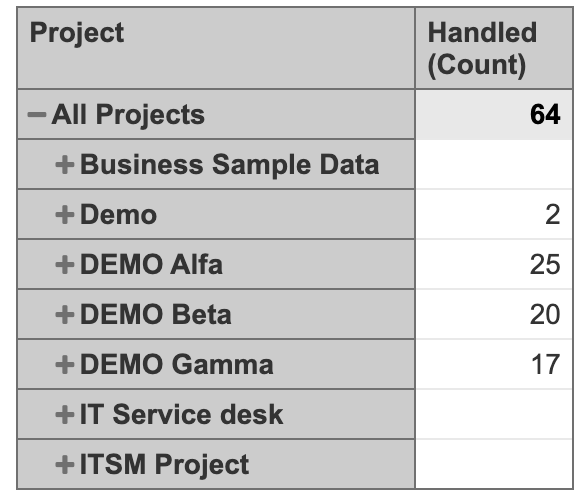# Link of a specific type

Hi,

I want to log the number of issues that are linked to a specific type of issue (“GCR”). The end goal is to display a ‘resolved vs handled’ graph (time on x-axis), where handled means that this type of link has been created.

Upon reading this tutorial I created the following:

name = “GCR”
issue_type = “GCR”
dimension = true

How do I convert this dimension into a usable measure?

Hi @MrDaggins,
You are on the right track with this!I would suggest updating your code with the additional line `multiple_values = true` that will recognize that in this dimension multiple values are stored.

Next check if you are importing this customfield as Properties.

When it is done and you have imported the customfield as property, you can create a calculated measure in Measure dimension that counts all issues where this property exists (NOT isEmpty()).

Handled (Count):

``````NonZero(
Count(
Filter(
Descendants([Issue].CurrentMember, [Issue].[Issue]),
NOT IsEmpty([Issue].CurrentHierarchyMember.get('GCR'))
AND
[Measures].[Issues created] > 0
)
)
)
``````

The example report would look like this:best,

Gerda //support@eazybi.com